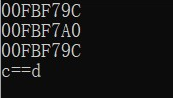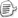class key1{
private:
int _val1;
};
class key2{
private:
int _val2;
};
class key3:public key1,public key2{
private:
int _val1;
};
int main(){
key3 a;
key1* b = &a;
key2* c = &a;
key3* d = &a;
cout << b <<endl<< c <<endl<< d<<endl;
if (c == d){
cout << "c==d" << endl;
}
else
cout << "c!=d" << endl;
return 0;
}posted on 2020-10-13 08:19  Kaniso_Vok  阅读(54)  评论(0编辑  收藏